Win up to 100% scholarship on Aakash BYJU'S JEE/NEET courses with ABNAT Win up to 100% scholarship on Aakash BYJU'S JEE/NEET courses with ABNAT

# JEE Main 2022 June 27 – Shift 1 Chemistry Question Paper with Solutions

JEE Main 2022 June 27th Shift 1 Chemistry question paper and solutions available here to help students check their answers written during the examination. These solutions will help them cross-check the right answers and predict the score in the exam. Also, you can get solutions for all JEE main 2022 question papers. Aspirants can try solving multiple JEE main question papers to enhance their preparation for the upcoming exams and improve their problem-solving skills. JEE main answer keys will also be a useful resource for the students who are solving previous year’s JEE main question papers.

## JEE Main 2022 27th June Morning Shift Chemistry Question Paper and Solutions

#### SECTION – A

Multiple Choice Questions: This section contains 20 multiple choice questions. Each question has 4 choices (1), (2), (3) and (4), out of which ONLY ONE is correct.

1. Given below are two statements : one is labelled as Assertion (A) and the other is labelled as Reason (R).

Assertion (A) : At 10°C, the density of a 5 M solution of KCl [atomic masses of K & Cl are 39 & 35.5 g mol–1 respectively], is ‘x’ g ml–1. The solution is cooled to –21°C. The molality of the solution will remain unchanged.

Reason (R) : The molality of a solution does not change with temperature as mass remains unaffected with temperature.

In the light of the above statements, choose the correct answer from the options given below.

(A) Both (A) and (R) are true and (R) is the correct explanation of (A).

(B) Both (A) and (R) are true but (R) is not the correct explanation of (A).

(C) (A) is true but (R) is false.

(D) (A) is false but (R) is true.

Sol. Density = ‘x’ gm ml–1

∴ molality,

$$\begin{array}{l}m=\frac{5\times1000}{\left [ x\left ( 1000 \right )-372.5 \right ]}=7.96\end{array}$$

≃ 8 m (Assuming x = 1)

∴ ΔTf = iKfm

Assuming complete dissociation of salt (100%)

(i = 2)

ΔTf = 2 × 1.86 × 8 ≃ 29.76

Hence, the solution does not freeze at –21°C. This means that molality of the solution won’t change as

x ≥ 1.

Statement (II) is also correct as molality is mass dependent and hence, does not change with temperature. However, as solvents are not mentioned, statement (I) can also be incorrect.

2. Based upon VSEPR theory, match the shape (geometry) of the molecules in List-I with the molecules in List-II and select the most appropriate option.

List-I List-II

(Shape) (Molecules)

(A) T-shaped (I) XeF4

(B) Trigonal planar (II) SF4

(C) Square planar (III) CIF3

(D) See-saw (IV) BF3

(A) (A)-(I), (B)-(II), (C)-(III), (D)-(IV)

(B) (A)-(III), (B)-(IV), (C)-(I), (D)-(II)

(C) (A)-(III), (B)-(IV), (C)-(II), (D)-(I)

(D) (A)-(IV), (B)-(III), (C)-(I), (D)-(II)

Sol.

(Shape) (Molecules)

(A) T-shaped (III) ClF3

(B) Trigonal planar (IV) BF3

(C) Square planar (I) XeF4

(D) See-saw (II) SF4

Hence, (B) is the correct option.

3. Match List-I with List-II

List-I

(A) Spontaneous process

(B) Process with ΔP = 0, ΔT = 0

(C) ΔHreaction

(D) Exothermic Process

List-II

(I) ΔH < 0

(II) ΔGT,P < 0

(III) Isothermal and isobaric process

(IV) [Bond energies of molecules in reactants] – [Bond energies of product molecules]

Choose the correct answer from the options given below :

(A) (A)-(III), (B)-(II), (C)-(IV), (D)-(I)

(B) (A)-(II), (B)-(III), (C)-(IV), (D)-(I)

(C) (A)-(II), (B)-(III), (C)-(I), (D)-(IV)

(D) (A)-(II), (B)-(I), (C)-(III), (D)-(IV)

Sol. Correct match is

1. Spontaneous (II) ΔGT,P < 0 process (Constant temperature and pressure condition)
2. Process with ΔP = 0, ΔT = 0 (III) Isothermal and isobaric process

(C) ΔHreaction (IV) [Bond energies of molecules in reactants – bond ene0072gies of product molecules]

(D) Exothermic process (I) ΔH < 0

Hence, the correct option is (B).

4. Match List-I with List-II

List-I List-II

(A) Lyophilic colloid (I) Liquid-liquid colloid

(B) Emulsion (II) Protective colloid

(C) Positively charged (III) FeCl3 + NaOH

colloid

(D) Negatively charged (IV) FeCl3 + hot water

colloid

Choose the correct answer from the options given below :

(A) (A)-(II), (B)-(I), (C)-(IV), (D)-(III)

(B) (A)-(III), (B)-(I), (C)-(IV), (D)-(II)

(C) (A)-(II), (B)-(I), (C)-(III), (D)-(IV)

(D) (A)-(III), (B)-(II), (C)-(I), (D)-(IV)

Sol. Correct match of List-I and List-II is:

1. Lyophilic colloid (II) Protective colloid
2. Emulsion (I) Liquid-liquid colloid

(C) Positively charged (IV) FeCl3 + hot water colloid (It forms a positively charged sol of Fe(OH)3)

(D) Negatively charged (III) FeCl3 + NaOH colloid (Negatively charged colloid is formed due to adsorption of OH ions on Fe(OH)3)

Hence, the correct option is (A).

5. Given below are two statements : one is labelled as Assertion (A) and the other is labelled as Reason (R).

Assertion (A) : The ionic radii of O2– and Mg2+ are same.

Reason (R) : Both O2– and Mg2+ are isoelectronic species.

In the light of the above statements, choose the correct answer from the options given below.

(A) Both (A) and (R) are true and (R) is the correct explanation of (A).

(B) Both (A) and (R) are true but (R) is not the correct explanation of (A).

(C) (A) is true but (R) is false.

(D) (A) is false but (R) is true.

Sol. Correct order of ionic radii:

O–2 > Mg+2

This is because among isoelectronic species, the size of anions are greater than the size of cations. Statement (II) is correct as both O–2 and Mg+2 are isoelectronic.

6. Match List-I with List-II.

List-I List-II

(A) Concentration of (I) Aniline

Gold ore

(B) Leaching of alumina (II) NaOH

(C) Froth stabiliser (III) SO2

(D) Blister copper (IV) NaCN

Choose the correct answer from the options given below.

(A) (A)-(IV), (B)-(III), (C)-(II), (D)-(I)

(B) (A)-(IV), (B)-(II), (C)-(I), (D)-(III)

(C) (A)-(III), (B)-(II), (C)-(I), (D)-(IV)

(D) (A)-(II), (B)-(IV), (C)-(III), (D)-(I)

Sol. List-I List-II

(A) Concentration of (IV) NaCN

Gold ore

(B) Leaching of alumina (II) NaOH

(C) Froth stabiliser (I) Aniline

(Aniline and cresols

are used as froth

stabilisers in froth

floatation process)

(D) Blister copper (III) SO2 (During self reduction process used in the formation of blister copper SO2 gas is evolved)

Hence (B) is most appropriate option.

7. Addition of H2SO4 to BaO2 produces:

(A) BaO, SO2 and H2O

(B) BaHSO4 and O2

(C) BaSO4, H2 and O2

(D) BaSO4 and H2O2

Sol.

$$\begin{array}{l}BaO_2\cdot8H_2O+H_2SO_4\rightarrow BaSO_4+H_2O_2+H_2O\end{array}$$

Hence, the correct option is (D)

8. BeCI2 reacts with LiAIH4 to give:

(A) Be + Li[AICI4] + H2

(B) Be + AIH3 + LiCI + HCI

(C) BeH2 + LiCI + AICI3

(D) BeH2 + Li[AICI4]

Sol.

$$\begin{array}{l}BeCl_2+LiAlH_4\rightarrow BeH_2+LiCl+AlCl_3\end{array}$$

The above reaction using LiAlH4 is an important preparation method for production of hydrides.

9. Match List-I with List-II.

List-I List-II

(Si-Compounds) (Si-Polymeric/other

Products)

(A) (CH3)4Si (I) Chain Silicone

(B) (CH3)Si(OH)3 (II) Dimeric Silicone

(C) (CH3)2Si(OH)2 (III) Silane

(D) (CH3)3Si(OH) (IV) 2D-Silicone

Choose the correct answer from the options given below:

(A) (A)-(III), (B)-(II), (C)-(I), (D)-(IV)

(B) (A)-(IV), (B)-(I), (C)-(II), (D)-(III)

(C) (A)-(II), (B)-(I), (C)-(IV), (D)-(III)

(D) (A)-(III), (B)-(IV), (C)-(I), (D)-(II)

Sol. List-I List-II

(Si-Compounds) (Si-Polymeric/other

Products)

(A) (CH3)4Si (III) Silane

(B) (CH3)Si(OH)3 (IV) 2D-Silicone

(C) (CH3)2Si(OH)2 (I) Chain Silicone

(D) (CH3)3Si(OH) (II) Dimeric Silicone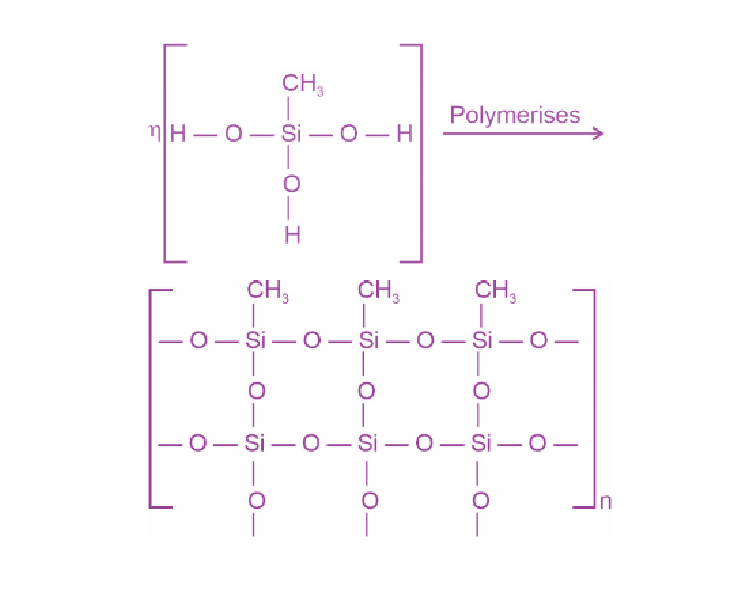2D-Silicone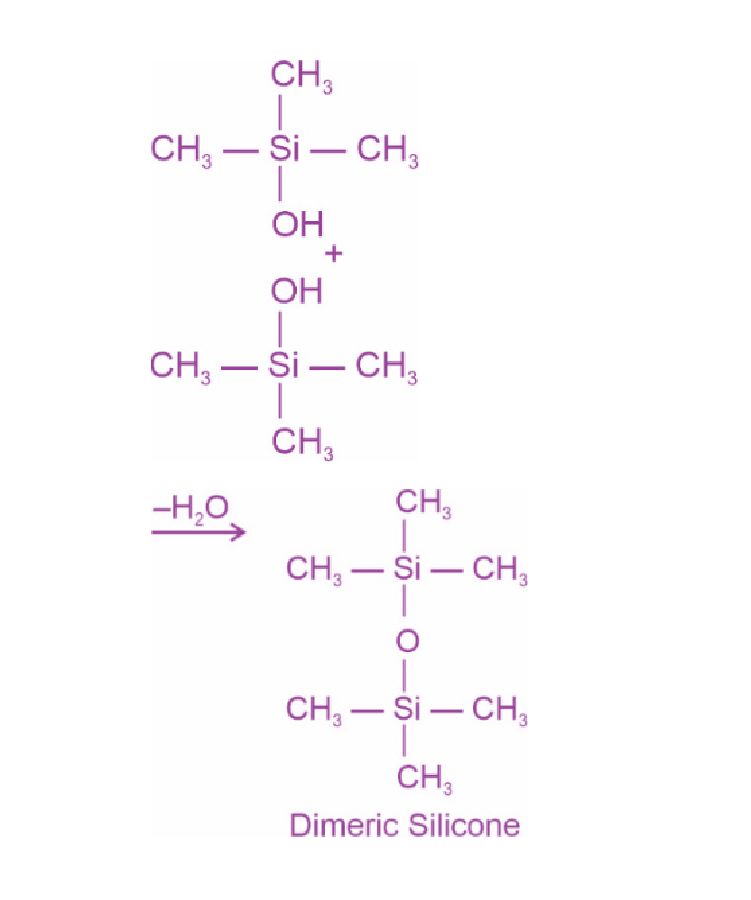10. Heating white phosphorus with conc. NaOH solution gives mainly:

(A) Na3P and H2O

(B) H3PO and NaH

(C) P(OH)3 and NaH2PO4

(D) PH3 and NaH2PO2

Sol. P4(white) + NaOH

→ PH3 + NaH2PO2 + H2O

11. Which of the following will have maximum stabilization due to crystal field?

(A) [Ti(H2O)6]3+

(B) [Co(H2O)6]2+

(C) [Co(CN)6]–3

(D) [Cu(NH3)4]2+

Sol. The given complexes are:

[Ti(H2O)6]3+, [Co(H2O)6]2+, [Co(CN)6]–3, [Cu(NH3)4]2+

CNis the strongest ligand among the given complexes CFSE value for the [Co(CN)6]–3 complex will be highest as it has d6 configuration with a CFSE value of –2.40 Δ0 + 2P, where P represents pairing energy and Δ0 represents splitting energy in octahedral field.

The value of Δ0 is high for cyanide complexes.

12. Given below are two Statements:

Statement I: Classical smog occurs in cool humid climate. It is a reducing mixture of smoke, fog and sulphur dioxide.

Statement II: Photochemical smog has components, ozone, nitric oxide, acrolein, formaldehyde, PAN etc.

In the light of the above statements, choose the most appropriate answer from the options given below.

(A) Both Statement I and Statement II are correct.

(B) Both Statement I and Statement II are incorrect.

(C) Statement I is correct but Statement II is incorrect.

(D) Statement I is incorrect but Statement II is correct

Sol. (I) Classical smog occurs in cool humid climate. It is a reducing mixture of smoke, fog and sulphur dioxide. This is a correct statement.

(II) This statement is also based on fact and is a correct statement.

13. Which of the following is structure of a separating funnel?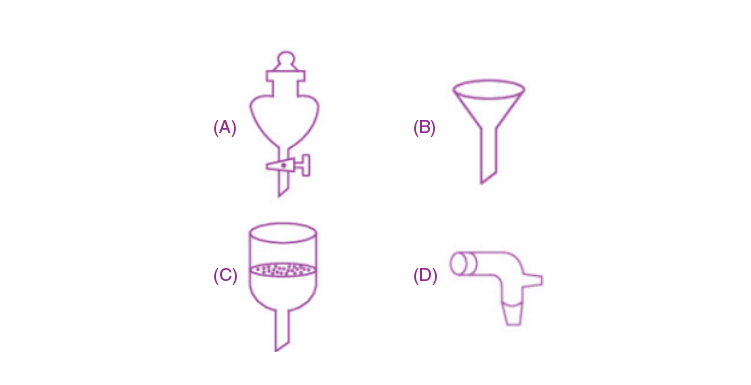Sol. The diagram is option (A) clearly represents separating funnel which is used to separate two immiscible liquids.

14. ‘A’ and ‘B’ respectively are:

$$\begin{array}{l}A\xrightarrow[\left ( 2 \right )Zn-H_2O]{(1)O_3}\end{array}$$
Ethane-1, 2-dicarbaldehyde + Glyoxal/Oxaldehyde

$$\begin{array}{l}B\xrightarrow[\left ( 2 \right )Zn-H_2O]{(1)O_3}5-\textup{oxohexanal}\end{array}$$

(A) 1-methylcyclohex-1, 3-diene & cyclopentene

(B) Cyclohex-1, 3-diene & cyclopentene

(C) 1-methylcyclohex-1, 4-diene & 1-methylcyclo-pent-ene

(D) Cyclohex-1, 3-diene & 1-methylcyclopent-1-ene

Sol.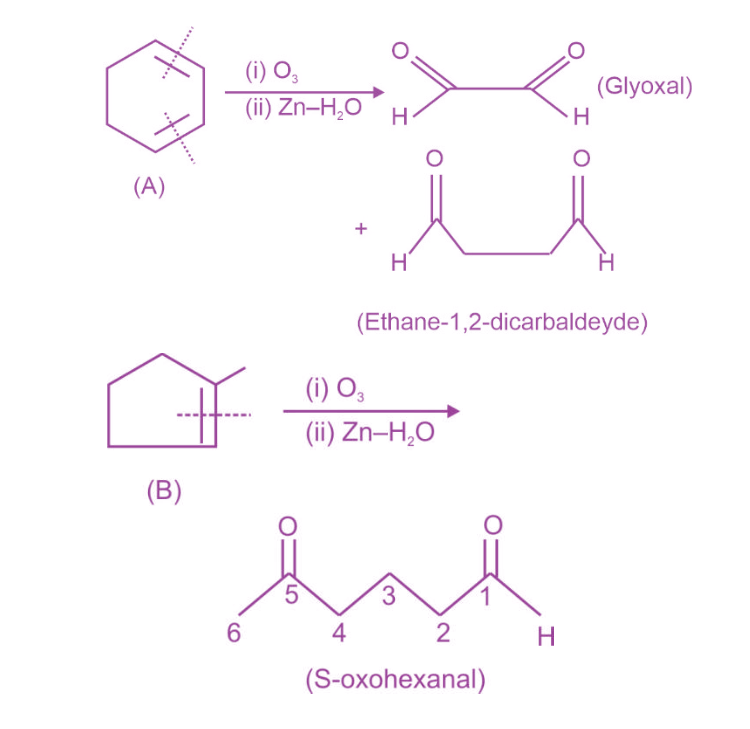(B) should be 1-methylcyclopent-1-ene.

15. The major product of the following reaction is: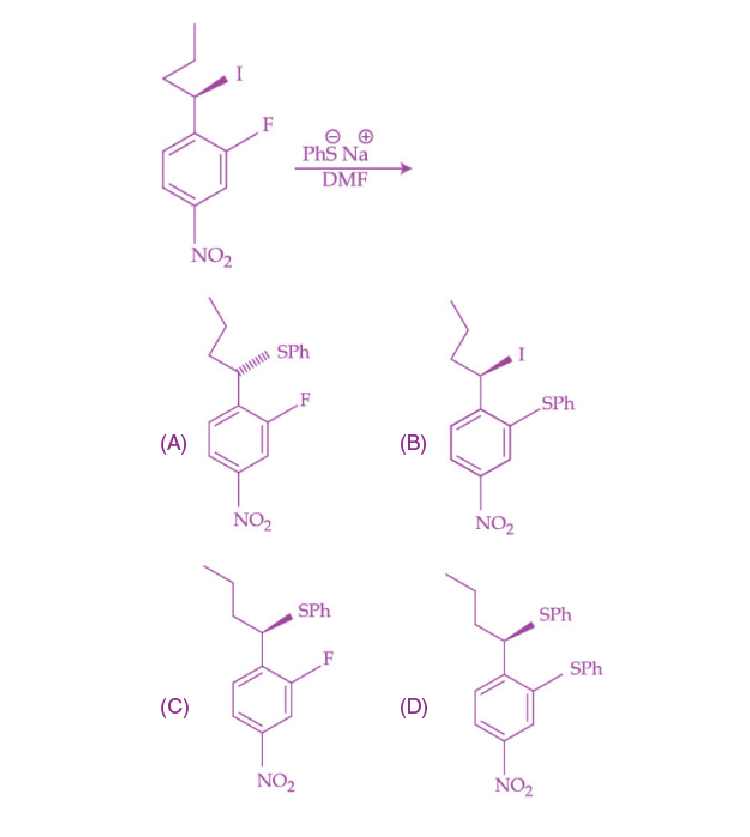Sol.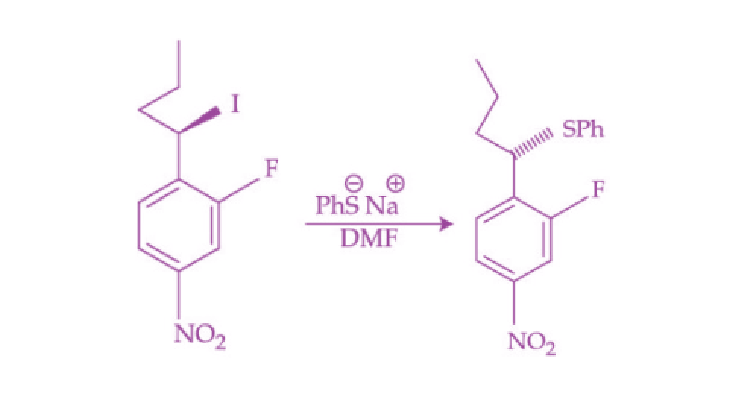Rate of SN2 >SN2 (AR)

16. Which of the following reactions will yield benzaldehyde as a product?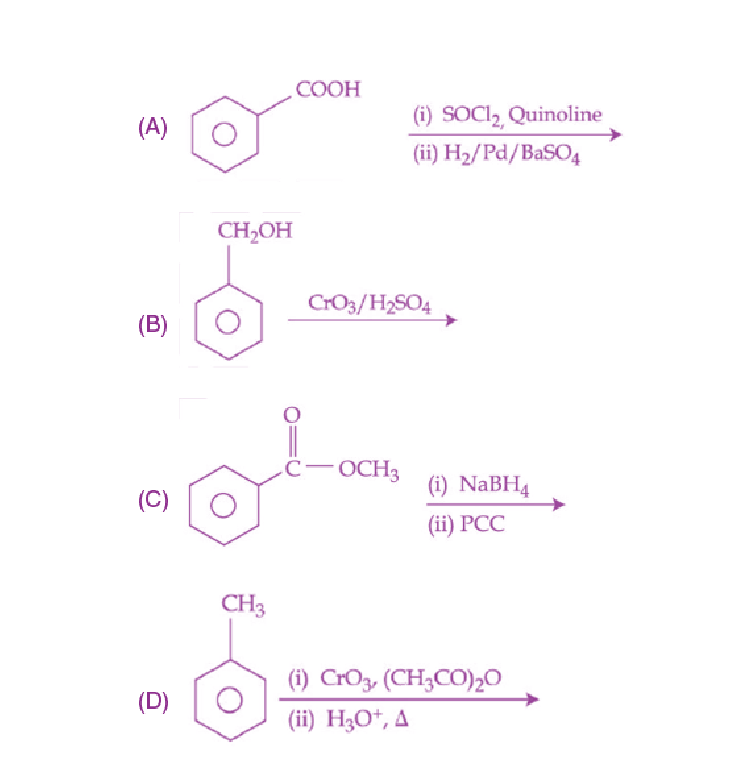(A) (B) and (C)

(B) (C) and (D)

(C) (A) and (D)

(D) (A) and (C)

Sol.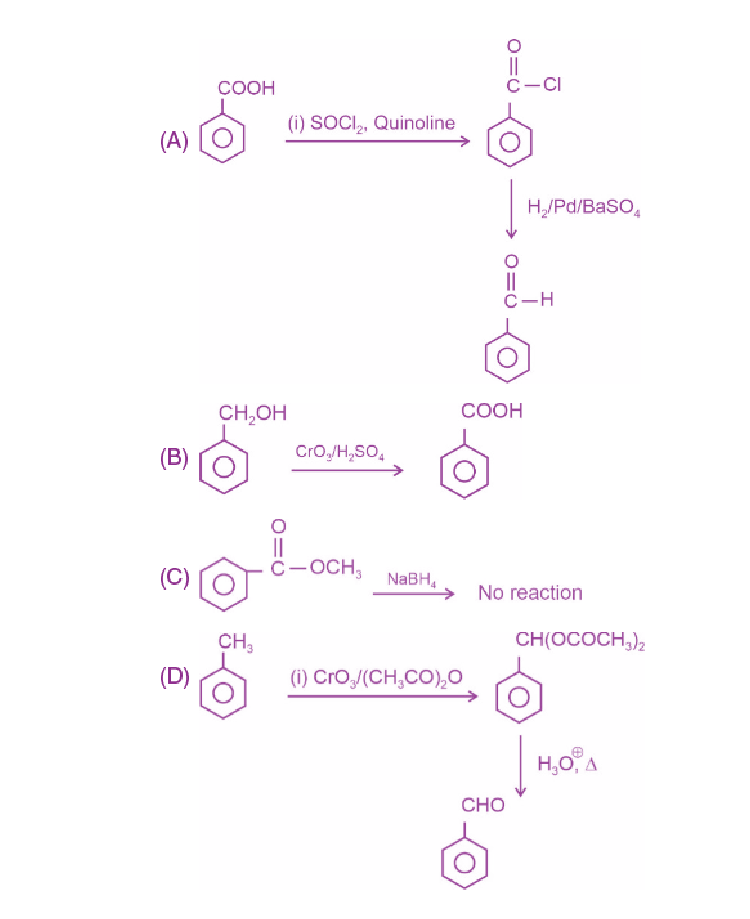17. Given below are two statements:

Statement-I : In Hofmann degradation reaction, the migration of only an alkyl group takes place from carbonyl carbon of the amide to the nitrogen atom.

Statement-II : The group is migrated in Hofmann degradation reaction to electron deficient atom.

In the light of the above statements, choose the most appropriate answer from the options given below:

(A) Both Statement I and Statement II are correct

(B) Both Statement I and Statement II are incorrect

(C) Statement I is correct but Statement II is incorrect

(D) Statement I is incorrect but Statement II is correct

In this degradation, the migration of the alkyl/aryl group occurs to the electron deficient nitrogen (nitrene).

Statement (I) is not absolutely correct as it mentions only the alkyl group, whereas migration of aryl groups may also occur depending on migratory aptitude.

Statement (II) is correct as migration occurs to electron deficient atom.

18. Match List-I with List-II

List-I List-II

(Polymer) (Used in)

(A) Bakelite (I) Radio and television cabinets

(B) Glyptal (II) Electrical switches

(C) PVC (III) Paints and Lacqures

(D) Polystyrene (IV) Water pipes

Choose the correct answer from the options given below:

(A) (A) –(II) (B) – (III), (C) – (IV), (D) – (I)

(B) (A) –(I) (B) – (II), (C) – (III), (D) – (IV)

(C) (A) –(IV) (B) – (III), (C) – (II), (D) – (I)

(D) (A) –(II) (B) – (III), (C) – (I), (D) – (IV)

Sol. List-I List-II

(Polymer) (Used in)

(A) Bakelite (II) Electrical switches

(B) Glyptal (III) Paints and Lacqures

(C) PVC (IV) Water pipes

(D) Polystyrene (I) Radio and television cabinets

Therefore, the correct option is (A).

19. L-isomer of a compound ‘A’ (C4H8O4) gives a positive test with [Ag(NH3)2]+. Treatment of ‘A’ with acetic anhydride yields triacetate derivative. Compound ‘A’ produces an optically active compound (B) and an optically inactive compound (C) on treatment with bromine water and HNO3 respectively. Compound (A) is: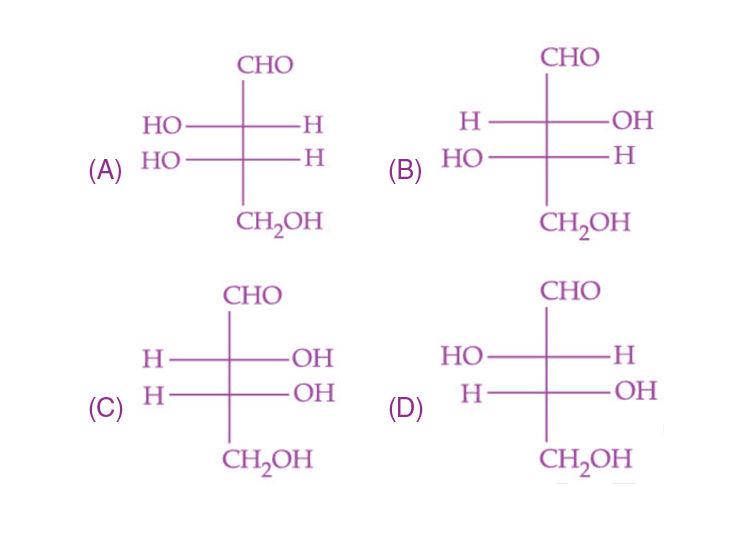Sol.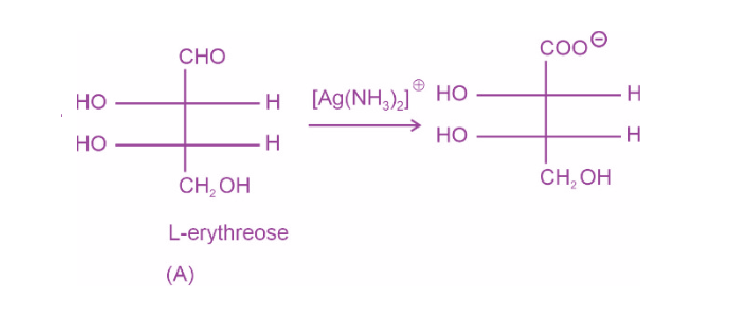When(A) is heated with acetic anhydride, acetylation occurs and –OH group is replaced by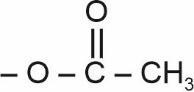and hence, triacetate is formed.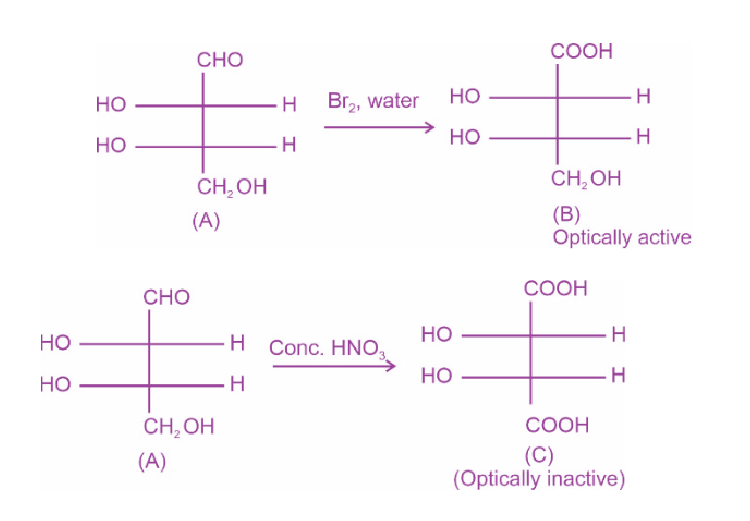20. Match List-I with List-II

List-I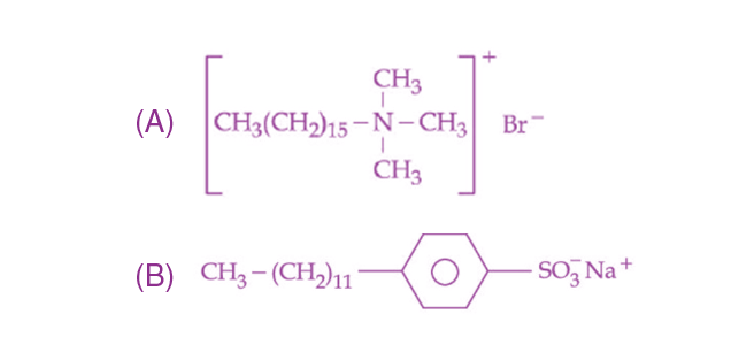(C) C17H35COONa++Na2CO3+Rosinate

(D) CH3(CH2)16COO(CH2CH2O)CH2CH2OH

List-II

(I) Dishwashing power

(II) Toothpaste

(III) Laundry soap

(IV) Hair conditional

Choose the correct answer from the options given below:

(A) (A) –(III) (B) – (II), (C) – (IV), (D) – (I)

(B) (A) –(IV) (B) – (II), (C) – (III), (D) – (I)

(C) (A) –(IV) (B) – (III), (C) – (II), (D) – (I)

(D) (A) –(III) (B) – (IV), (C) – (I), (D) – (II)

Sol.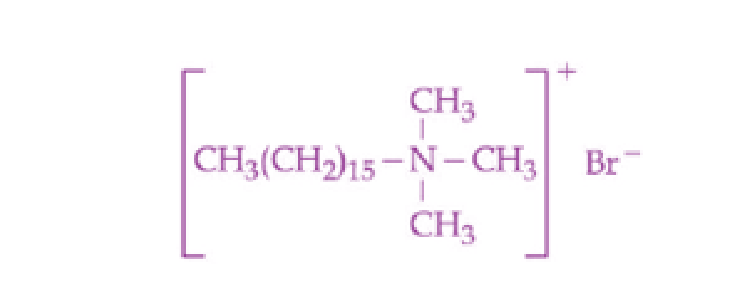→ Cationic detergent

(Hair conditioner)

(B)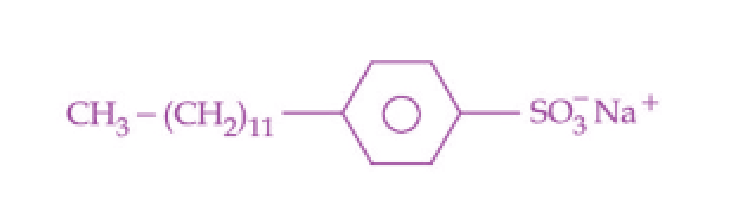→ Toothpaste

(Anionic detergent)

(C) C17H35COONa++Na2CO3+Rosinate

→ Laundry soap

(D) CH3(CH2)16COO(CH2CH2O)CH2CH2OH

→ Dishwashing powder

#### SECTION – B

Numerical Value Type Questions: This section contains 10 questions. In Section B, attempt any five questions out of 10. The answer to each question is a NUMERICAL VALUE. For each question, enter the correct numerical value (in decimal notation, truncated/rounded-off to the second decimal place; e.g. 06.25, 07.00, –00.33, –00.30, 30.27, –27.30) using the mouse and the on-screen virtual numeric keypad in the place designated to enter the answer.

1. Metal deficiency defect is shown by Fe0.93O. In the crystal, some Fe2+ cations are missing and loss of positive charge is compensated by the presence of Fe3+ ions. The percentage of Fe2+ ions in the Fe0.93O crystals is ______. (Nearest integer)

Sol. Fe0.93O

Let the number of O–2 ions be 100

and the number of Fe+2 ions be X

The number of Fe+3 ions be (93 – X)

∴ X(2) + (93 – X)3 = 200

279 – X = 200

X = 79

∴ % of Fe+2 ions

$$\begin{array}{l}=\frac{79}{93}\times 100\end{array}$$

≃ 85%

2. If the uncertainty in velocity and position of a minute particle in space are, 2.4 × 10–26 (m s–1) and 10–7 (m), respectively. The mass of the particle in g is _____. (Nearest integer)

(Given : h = 6.626 × 10–34 Js)

Sol.

$$\begin{array}{l}\Delta v=2.4\times10^{-26}~\text{ms}^{-1}\end{array}$$
$$\begin{array}{l}\Delta x=10^{-7}~\text{m}\end{array}$$
$$\begin{array}{l}\therefore m\geq\frac{h}{4\pi\left ( \Delta x \right )\left ( \Delta v \right )}\end{array}$$
$$\begin{array}{l}\geq \frac{6.626\times10^{-34}}{4\times3.14\times\left ( 10^{-7} \right )\left ( 2.4 \right )\times10^{-26}}\end{array}$$
$$\begin{array}{l}\geq \frac{6.626\times10^{-1}}{4\times2.4\times3.14}\end{array}$$

≥ 0.02198 kg

∴ Mass of the particle ≃ 22 g

3. 2 g of a non-volatile non-electrolyte solute is dissolved in 200 g of two different solvents A and B whose ebullioscopic constants are in the ratio of 1 : 8. The elevation in boiling points of A and B are in the ratio

$$\begin{array}{l}\frac{x}{y}\end{array}$$
(x : y). The value of y is ______. (Nearest Integer)

Sol. ΔTb = kbm

$$\begin{array}{l}\frac{\left ( \Delta T_b \right )_A}{\left ( \Delta T_b \right )_B}=\frac{\left ( k_b \right )_A}{\left ( k_b \right )_B}\end{array}$$
$$\begin{array}{l}=\frac{1}{8}=\frac{x}{y}\end{array}$$
$$\begin{array}{l}\therefore y=8\end{array}$$

4.

$$\begin{array}{l}2NOCl(g)\rightleftharpoons2NO(g) + Cl_2(g)\end{array}$$

In an experiment, 2.0 moles of NOCl was placed in a one-litre flask and the concentration of NO after equilibrium established, was found to be 0.4 mol/ L. The equilibrium constant at 30°C is _____ × 10–4.

Sol.

$$\begin{array}{l}2NOCl(g)\rightleftharpoons2NO(g) + Cl_2(g)\end{array}$$

t = 0 2

t = teq 2 – 0.4 0.4 0.2

$$\begin{array}{l}k_c=\frac{\left ( 0.2 \right )\times\left ( 0.4 \right )^2}{\left ( 1.6 \right )^2}\end{array}$$
$$\begin{array}{l}=\frac{0.2}{16}=\frac{1}{8}\times10^{-1}\end{array}$$
$$\begin{array}{l}=0.125\times10^{-1}\end{array}$$
$$\begin{array}{l}=125\times10^{-4}\end{array}$$

5. The limiting molar conductivities of NaI, NaNO3 and AgNO3 are 12.7, 12.0 and 13.3 mS m2 mol–1, respectively (all at 25°C). The limiting molar conductivity of Agl at this temperature is ____ mS m2 mol–1.

Sol.

$$\begin{array}{l}\Lambda_m^0\left ( Agl \right )=\Lambda_m^0\left ( Nal \right )+\Lambda_m^0\left ( AgNO_3 \right )-\Lambda_m^0\left ( NaNO_3 \right )\end{array}$$

= 12.7 + 13.3 – 12.0

= 26 – 12

= 14 mS m2 mol–1

6. The rate constant for a first order reaction is given by the following equation :

$$\begin{array}{l}Ink=33.24-\frac{2.0\times10^4K}{T}\end{array}$$

The activation energy for the reaction is given by ______ kJ mol–1. (In nearest integer)

(Given : R = 8.3 J K–1 mol–1)

Sol.

$$\begin{array}{l}Ink=33.24-\frac{2\times10^4}{T}\end{array}$$

$$\begin{array}{l}\frac{E_a}{R}=2\times10^4\end{array}$$

Ea = 2 × 104 × 8.3

= 166 kJ/mol

7. The number of statement(s) correct from the following for Copper (at. no. 29) is/are ______.

(A) Cu(II) complexes are always paramagnetic

(B) Cu(I) complexes are generally colourless

(C) Cu(I) is easily oxidized

(D) In Fehling solution, the active reagent has Cu(I)

Sol. (A) Cu(II) complexes are always paramagnetic as they have one unpaired electron due to d9 configuration of Cu(II)

(B) Cu(I) complexes are generally colourless due to d10 configuration.

(C) Cu(I) is easily oxidised to Cu+2 in aqueous solution

2Cu+ → Cu+2 + Cu

Cu+1 disproportionates to Cu+2 and Cu

(

$$\begin{array}{l}E_\textup{cell}^\circ>0\end{array}$$
for this cell reaction in aqueous solution)

In Fehling’s solution, active reagent has Cu(II) which is reduced to Cu(I) on reaction with aldehydes.

Hence (D) statement is incorrect.

8. Acidified potassium permanganate solution oxidises oxalic acid. The spin-only magnetic moment of the manganese product formed from the above reaction is ______ B.M.

(Nearest Integer)

Sol.

$$\begin{array}{l}KMnO_4\left ( \textup{acidic~medium} \right)+H_2C_2O_4\rightarrow CO_2+Mn^{2+}\end{array}$$

Mn+2 has 5 unpaired electrons

∴ Spin only magnetic moment

$$\begin{array}{l}=\sqrt{5\left ( 5+2 \right )}\end{array}$$
$$\begin{array}{l}=\sqrt{5\times7}\end{array}$$
$$\begin{array}{l}=\sqrt{35}\end{array}$$
$$\begin{array}{l}\simeq 5.92~\text{B.M.}\end{array}$$
$$\begin{array}{l}\simeq 6~\text{B.M.}\end{array}$$

9. Two elements A and B which form 0.15 moles of A2B and AB3 type compounds. If both A2B and AB3 weigh equally, then the atomic weight of A is _____ times of atomic weight of B.

Sol. Mole of A2B = moles of AB3

$$\begin{array}{l}\frac{W}{2A+B}=\frac{W}{A+3B}\end{array}$$

A + 3B = 2A + B

2B = A

Atomic weight of A is 2 times that of B.

10. Total number of possible stereoisomers of dimethyl cyclopentane is _______.

Sol. Position of methyl groups not mentioned.

### Download PDF of JEE Main 2022 June 27 Shift 1 Chemistry Paper & Solutions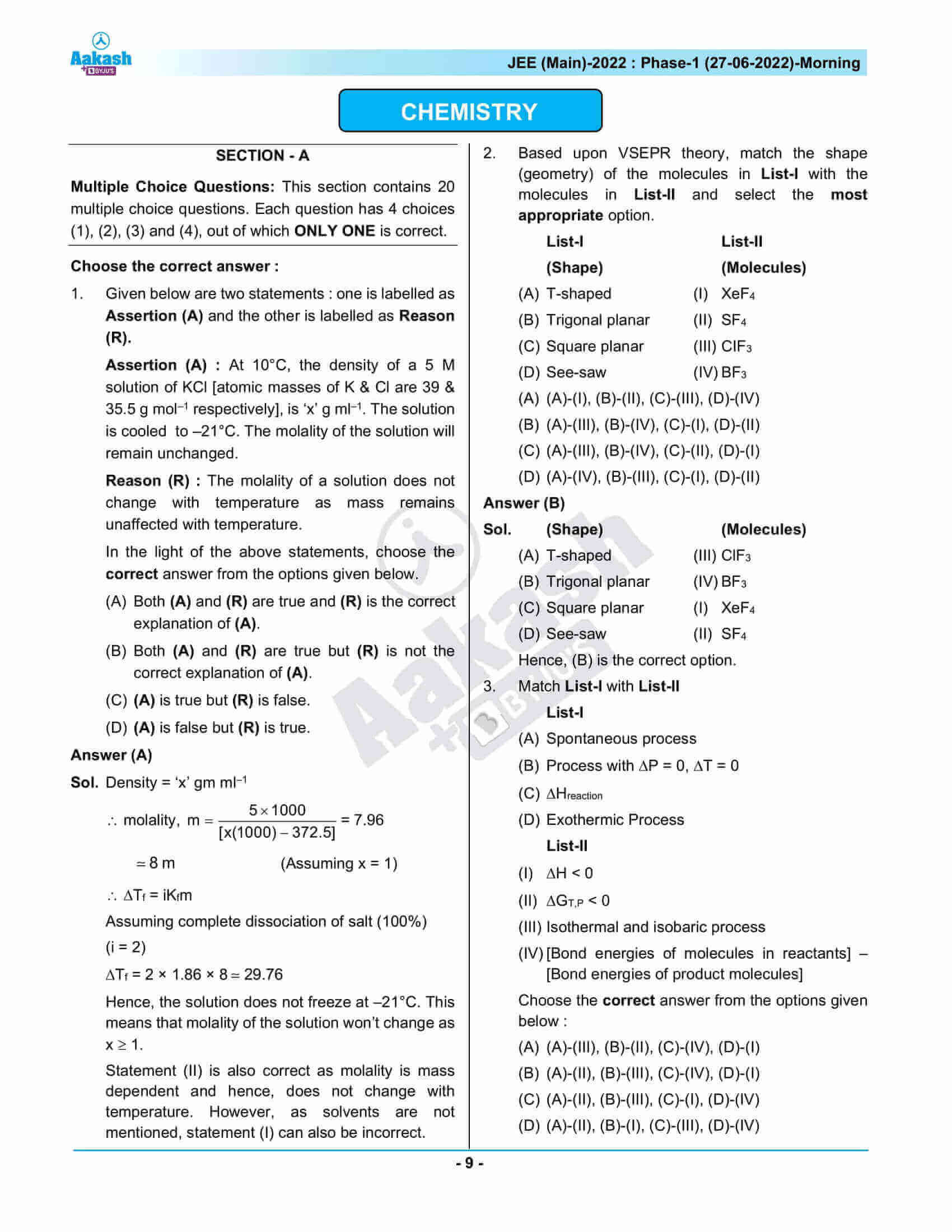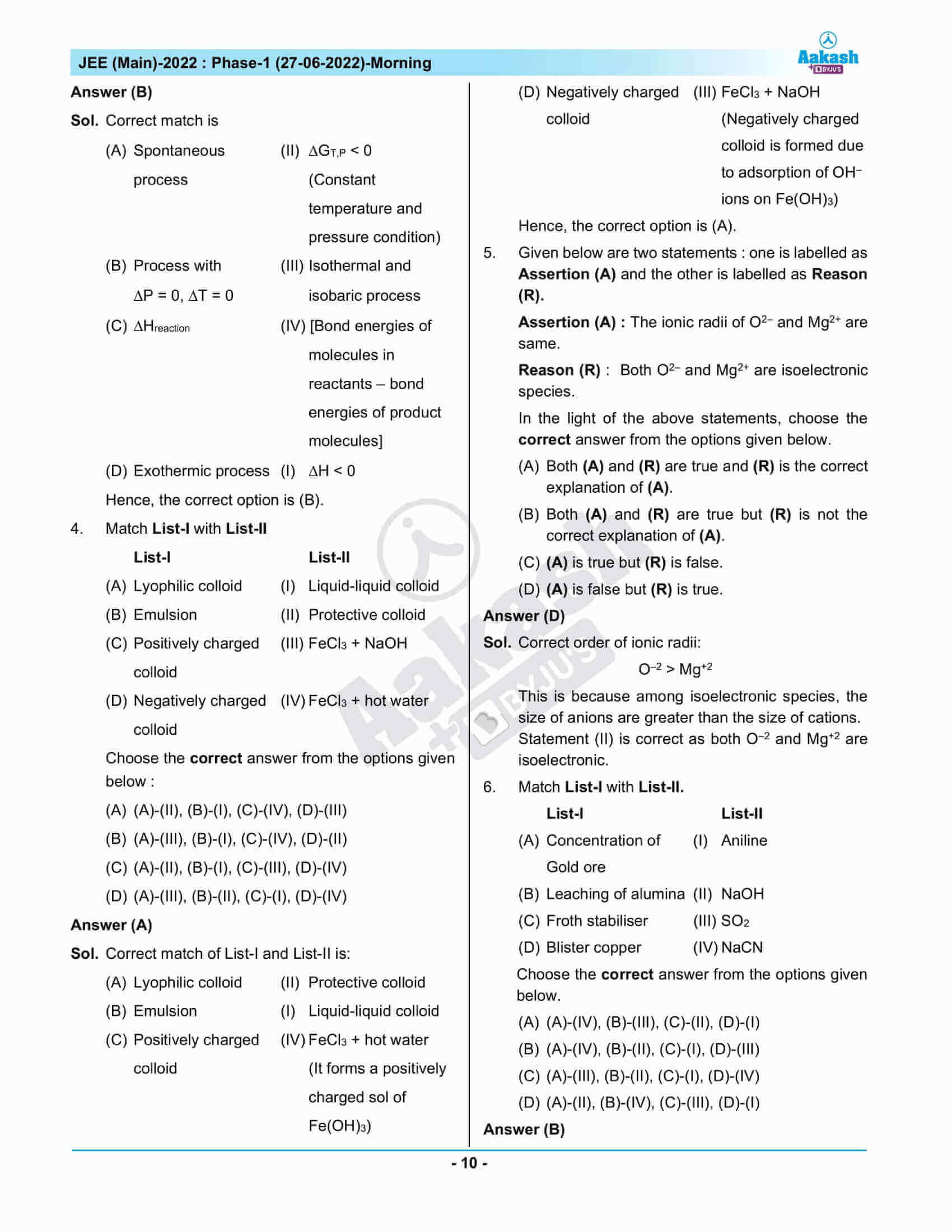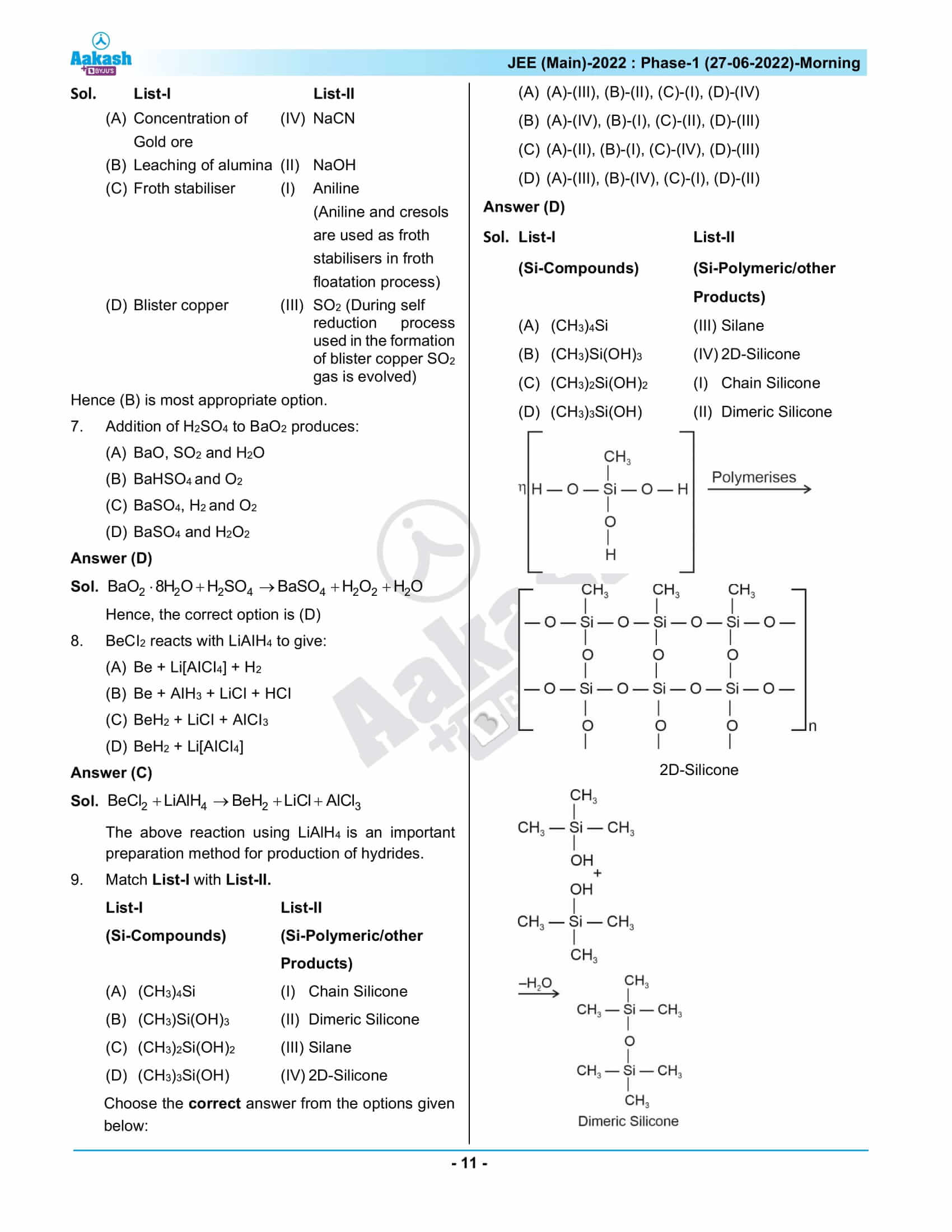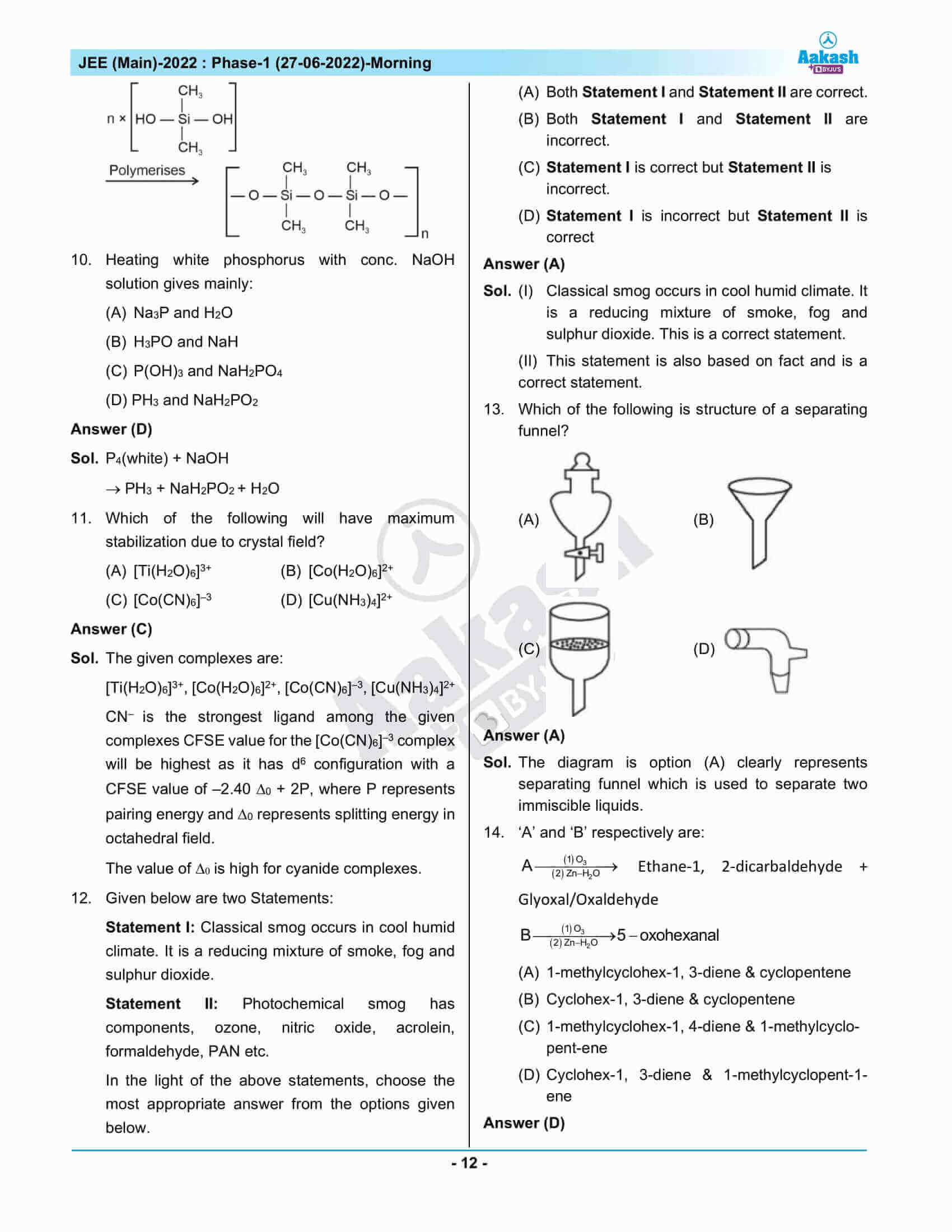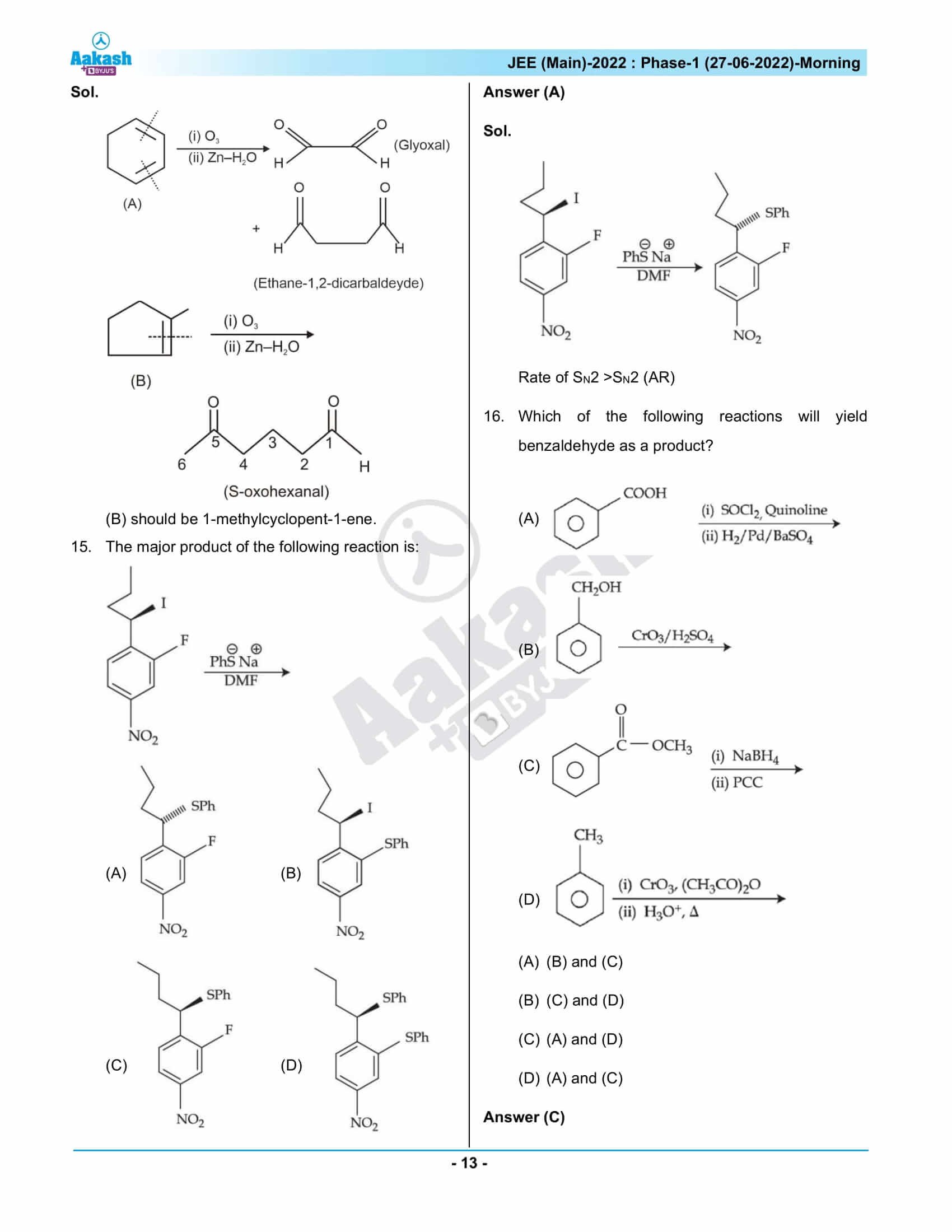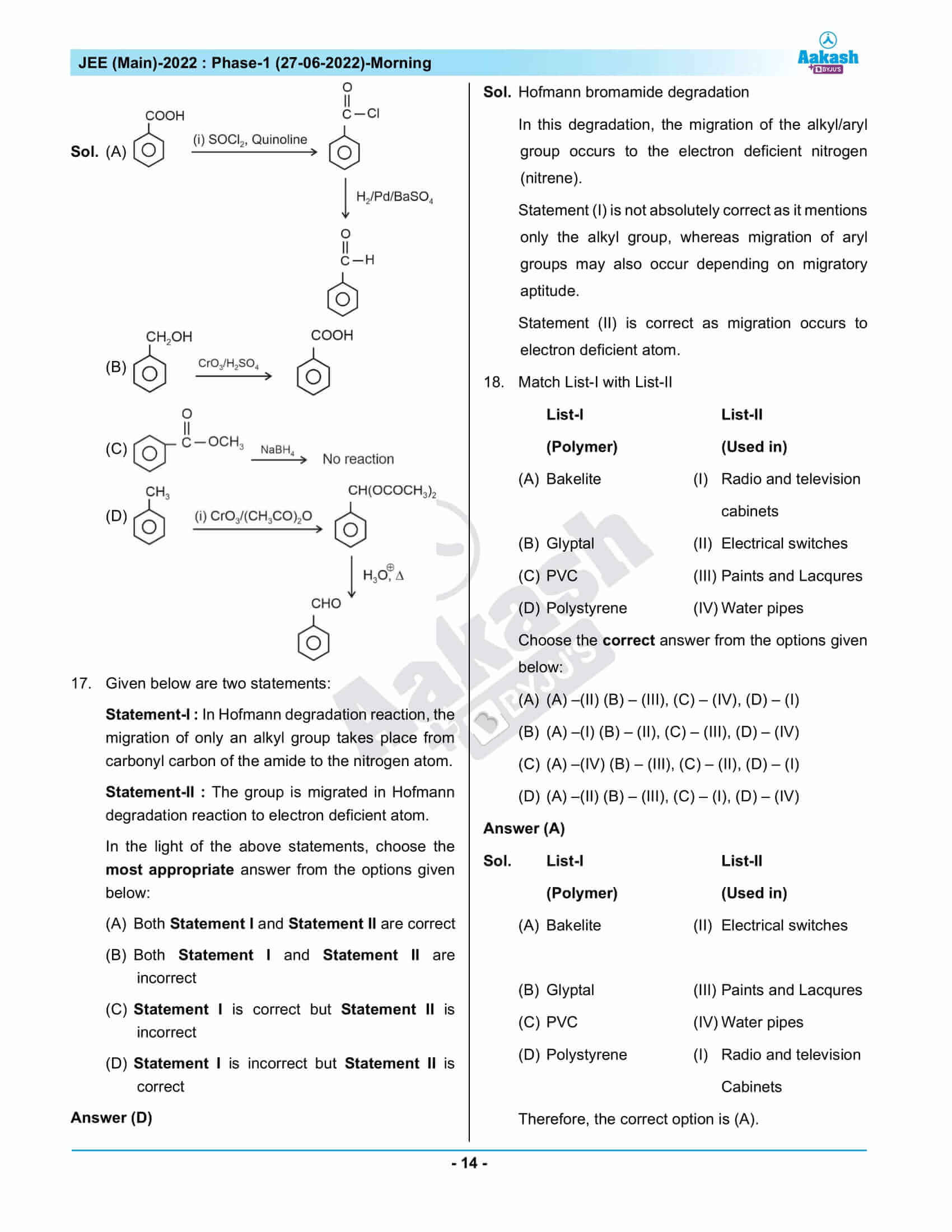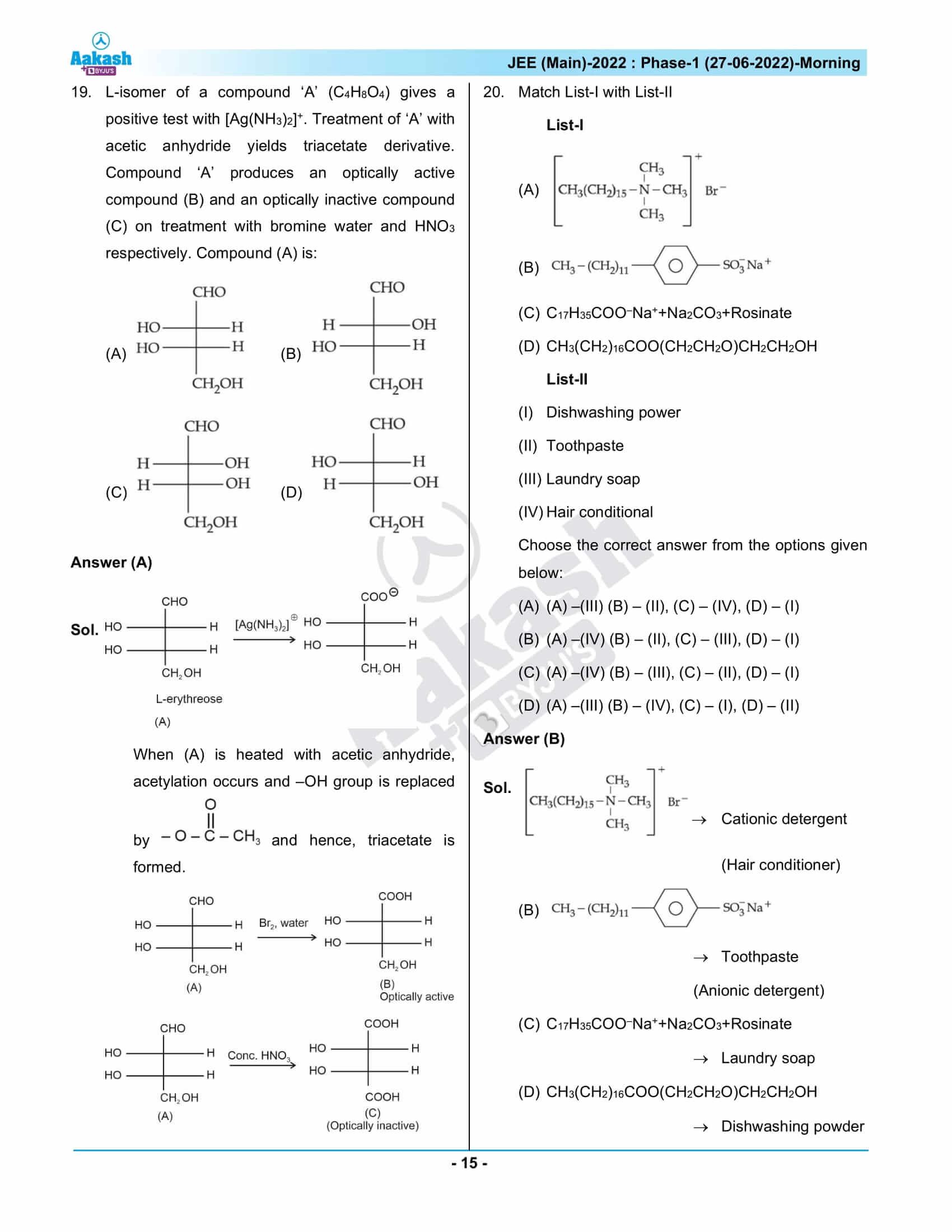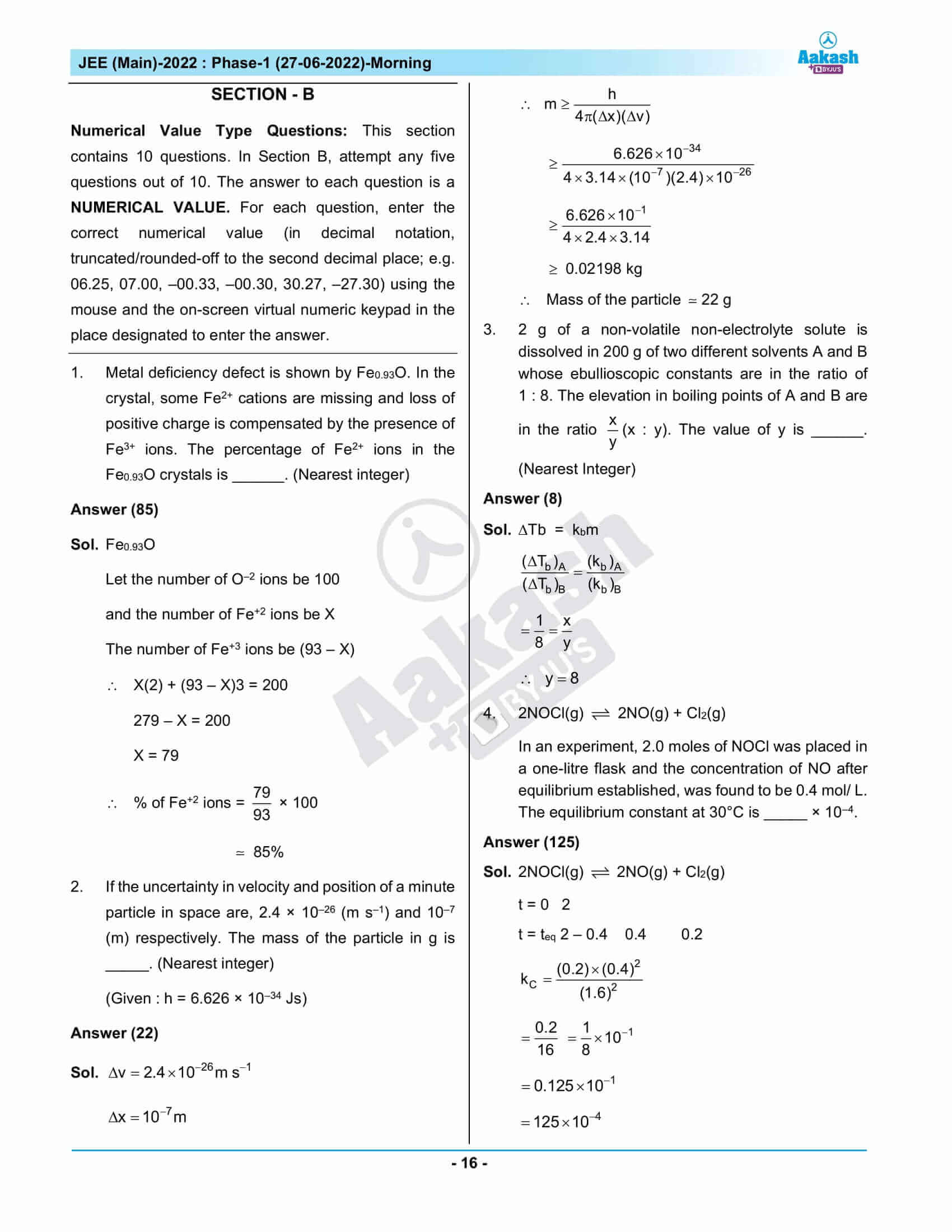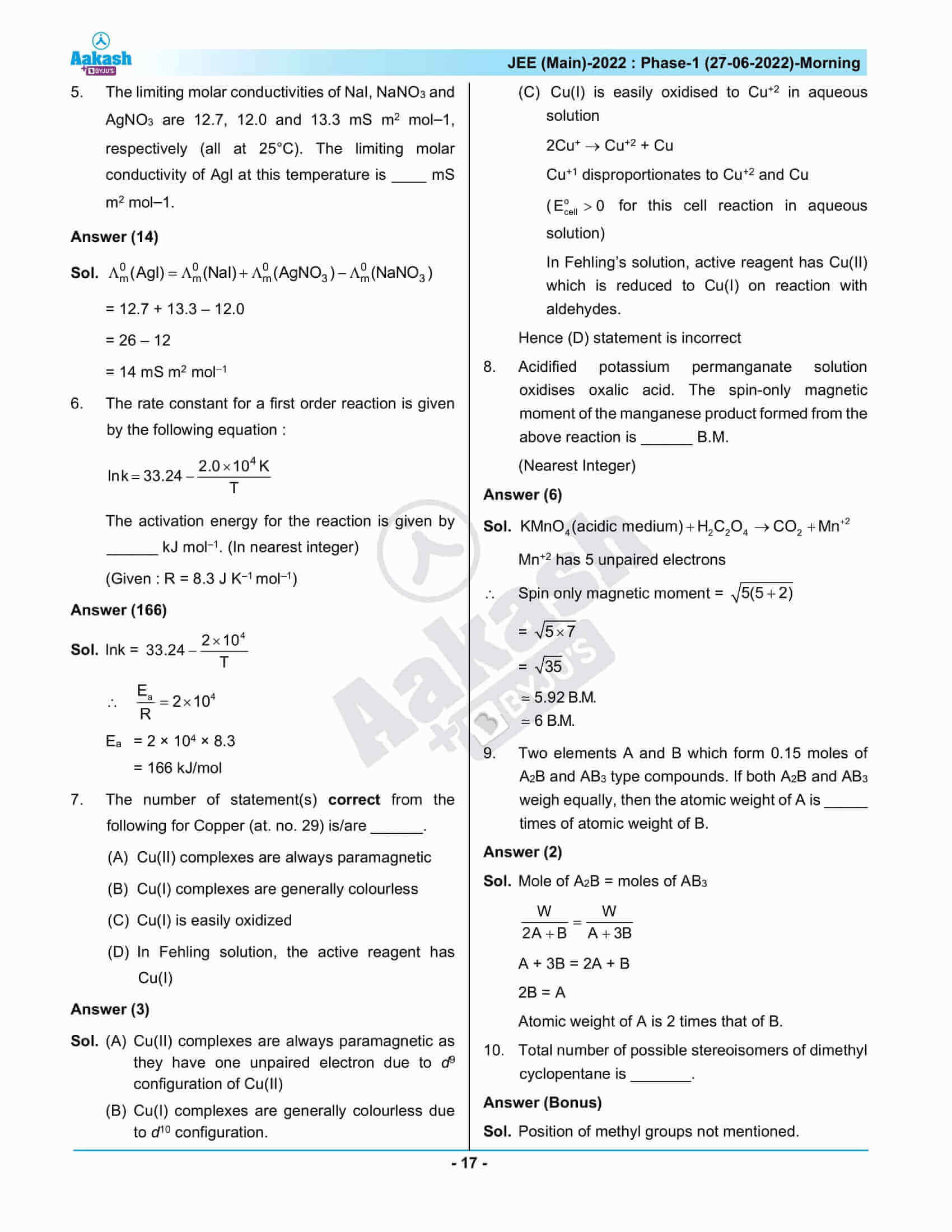## JEE Main 2022 Question Paper with Solutions – June 27th Shift 1

### JEE Main 2022 June 27 Shift 1 Question Paper – Physics Solutions### JEE Main 2022 June 27 Shift 1 Question Paper – Chemistry Solutions### JEE Main 2022 June 27 Shift 1 Question Paper – Maths Solutions## Frequently Asked Questions – FAQs

Q1

### How was the JEE Main 2022 Shift 1 June 27 Chemistry question paper?

JEE Main 2022 Shift 1 June 27 Chemistry question paper had moderate to a difficult level of questions. There were 13 medium and 7 easy questions and 3 questions were difficult as per the memory-based question paper.

Q2

### What is the overall difficulty level of the JEE Main 2022 June 27 Shift 1 Chemistry question paper?

The overall difficulty level of the JEE Main 2022 June 27 Shift 1 Chemistry question paper is 1.83 out of 3.

Q3

### What type of questions were asked from Hydrocarbons in the JEE Main 2022 June 27 Shift 1 Chemistry question paper?

Medium difficulty level questions were asked from Hydrocarbons in the JEE Main 2022 June 27 Shift 1 Chemistry question paper.

Q4

### What is the difficulty level of questions asked from Haloalkanes and Haloarenes in the JEE Main 2022 Shift 1 June 27 Chemistry question paper?

The questions asked from Haloalkanes and Haloarenes in the JEE Main 2022 Shift 1 June 27 Chemistry question paper were quite difficult.

Q5

### Is there a choice in section B of the JEE Main 2022 June 27 Chemistry Shift 1 question paper?

Yes. There is a choice in section B. Students need to attempt only 5 out of the 10 questions.